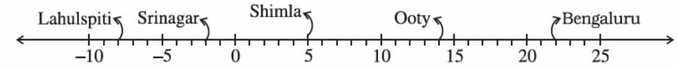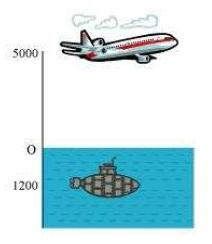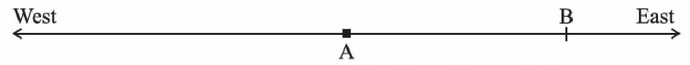# NCERT Solutions Class-7 Maths chapter-1 Exercise-1.1

## Integers Exercise-1.1

NCERT Solutions Class-7 Maths chapter-1 Integer Exercise-1.1 is prepared by academic team of Physics Wallah all the questions of NCERT text book are solved step by step with proper and detail solutions explaining each and every questions . For More and additional questions of CBSE class 7 maths you can go to class 7 maths sections. NCERT class 7 Maths Solutions is the best way to enhanced your mathematics skill. And Physics Wallah practice worksheet & question bank will help you a lot.

### Solutions of Chapter Integeres Exercise-1.1

Question 1:

Following number line shows the temperature in degree celsius (°C) at different places on a particular day.(a) Observe this number line and write the temperature of the places marked on it.

(b) What is the temperature difference between the hottest and the coldest places among the above?

(c) What is the temperature difference between Lahulspiti and Srinagar?

(d) Can we say temperature of Srinagar and Shimla taken together is less than the temperature at Shimla? Is it also less than the temperature at Srinagar?

(a) By observing the given data, the temperatures of these cities are as follows.

Lahulspiti : −8°C

Srinagar : −2°C

Shimla : 5°C

Ooty : 14°C

Bangalore : 22°C

(b) Temperature at the hottest place, i.e., Bangalore = 22°C

Temperature at the coldest place, i.e., Lahulspiti = −8°C

Temperature difference = 22°C − (−8°C)

= 30°C

Therefore, the temperature difference between the hottest and the coldest places is 30ºC.

(c) Temperature at Lahulspiti = −8°C

Temperature at Srinagar = −2°C

Temperature difference = −2°C − (−8°C)

= 6°C

Therefore, the temperature difference between Lahulspiti and Srinagar is 6ºC.

(d) Temperature at Srinagar = −2°C

Temperature at Shimla = 5°C

Temperature of Srinagar and Shimla taken together = −2°C + 5°C

= 3°C

3°C <  5°C

3°C <  Temperature of Shimla Yes, the temperature of Srinagar and Shimla taken together is less than the temperature of Shimla. However, 3°C < −2°C

Hence, the temperature of Srinagar and Shimla taken together is not less than the temperature of Srinagar.

Question 2:

In a quiz, positive marks are given for correct answers and negative marks are given for incorrect answers. If jack’s scores in five successive rounds were 25, − 5, − 10, 15 and 10, what was his total at the end?

Jack’s scores in five successive rounds are 25, −5, −10, 15, and 10. Total score of Jack at the end will be the sum of these scores.

Therefore, Jack’s total score at the end = 25 − 5 − 10 + 15 + 10 = 35

Question 3:

At Srinagar temperature was − 5°C on Monday and then it dropped by 2°C on Tuesday.

What was the temperature of Srinagar on Tuesday? On Wednesday, it rose by 4°C. What was the temperature on this day?

Temperature on Monday = −5°C

Temperature on Tuesday = Temperature on Monday −2°C

= −5°C − 2°C = −7°C

Temperature on Wednesday = Temperature on Tuesday + 4°C

= −7°C + 4°C = −3°C

Therefore, the temperature on Tuesday and Wednesday was −7ºC and −3ºC respectively.

Question 4:

A plane is flying at the height of 5000 m above the sea level. At a particular point, it is exactly above a submarine floating 1200 m below the sea level. What is the vertical distance between them?Height of plane = 5000 m

Depth of submarine = −1200 m

Distance between plane and submarine = 5000 m − (−1200) m

= 5000 m + 1200 m = 6200 m

Question 5:

Mohan deposits Rs 2,000 in his bank account and withdraws Rs 1,642 from it, the next day. If withdrawal of amount from the account is represented by a negative integer, then how will you represent the amount deposited? Find the balance in Mohan’s account after the withdrawal.

Since the amount withdrawn is represented by a negative integer, the amount deposited will be represented by a positive integer.

Amount deposited = Rs 2000

Amount withdrawn = −Rs 1642

Balance in Mohan’s account = Money deposited + Money withdrawn

= 2000 + (−1642) = 2000 − 1642 = 358

Therefore, balance in Mohan’s account after withdrawal is Rs 358.

Question 6:

Rita goes 20 km towards east from a point A to the point B. From B, she moves 30 km towards west along the same road. If the distance towards east is represented by a positive integer then, how will you represent the distance travelled towards west? By which integer will you represent her final position from A?Since the distance towards east is represented by a positive integer, the distance travelled towards west will be represented by a negative integer.

Distance travelled in east direction = 20 km

Distance travelled in west direction = −30 km

Distance travelled from A = 20 + (−30) = −10 km

Therefore, we will represent the distance travelled by Rita from point A by a negative integer, i.e., −10 km (i.e., Rita is now in west direction).

Question 7:

In a magic square each row, column and diagonal have the same sum. Check which of the following is a magic square.It can be observed that in square (i), every row and column add up to give 0. However, the sum of one of its diagonals is not 0.

As − 4 − 2 = −6 ≠ 0,

Therefore, (i) is not a magic square.

Similarly, in square (ii), each row, column, and diagonal add up to give −9. Therefore,

(ii) is a magic square.

Question 8:

Verify a − (−b) = a + b for the following values of a and b.

(i) a = 21, b = 18

(ii) a = 118, b = 125

(iii) a = 75, b = 84

(iv) a = 28, b = 11

(i) a = 21, b = 18

a − (−b) = 21 − (−18) = 21 + 18 = 39

a + b = 21 + 18 = 39

∴a − (−b) = a + b = 39

(ii) a = 118, b = 125

a − (−b) = 118 − (−125) = 118 + 125 = 243

a + b = 118 + 125 = 243

∴a − (−b) = a + b = 243

(iii) a = 75, b = 84

a − (−b) = 75 − (−84) = 75 + 84 = 159

a + b = 75 + 84 = 159

∴ a − (−b) = a + b = 159

(iv) a = 28, b = 11

a − (−b) = 28 − (−11) = 28 + 11 = 39

a + b = 28 + 11 = 39

∴ a − (−b) = a + b = 39

Question 9:  Use the sign of >, < or = in the box to make the statements true:

(a) (-8) + (-4) ….. (-8) - (-4)

Solution: We have,

(-8) + (-4)

= -8 – 4

= - 12

Also,

(-8) – (-4)

= - 8 + 4

= - 4

Hence,

-12 < - 4

(-8) + (-4) < (-8) - (-4)

(b) (-3) + 7 – (19) ….. 15 – 8 + (-9)

Solution: We have,

(-3) + 7 – (19)

= - 3 + 7 – 19

= - 22 + 7

= - 15

Also,

15 – 8 + (-9)

= 15 – 8 – 9

= - 2

Hence,

- 15 < - 2

(-3) + 7 – (19) < 15 – 8 + (-9)

(c) 23 – 41 + 11 ….. 23 – 41 – 11

Solution: We have,

23 - 41 + 11

= - 7

Also,

23 – 41 – 11

= 23 – 52

= - 29

Hence,

- 7 > - 29

23 – 41 + 11 > 23 – 41 – 11

(d) 39 + (-24) – (15) ….. 36 + (-52) – (-36)

Solution: We have,

39 + (-24) – (15)

= 39 – 24 - 15

= 39 – 39

= 0

Also,

36 + (-52) – (- 36)

= 36 – 52 + 36

= 72 – 52

= 20

Hence,

0 < 20

39 + (-24) – (15) < 36 + (-52) – (-36)

(e) – 231 + 79 + 51 ….. – 399 + 159 + 81

Solution: We have,

- 231 + 79 + 51

= - 231 + 130

= - 101

Also,

- 399 + 159 + 81

= - 399 + 240

= - 159

Hence,

- 101 > - 159

– 231 + 79 + 51 >